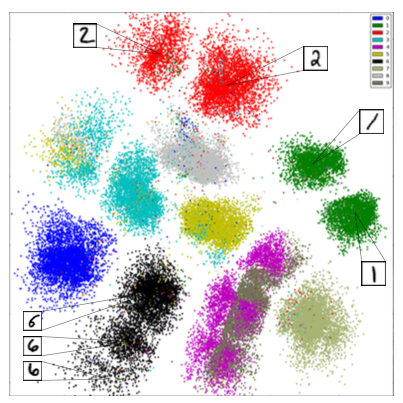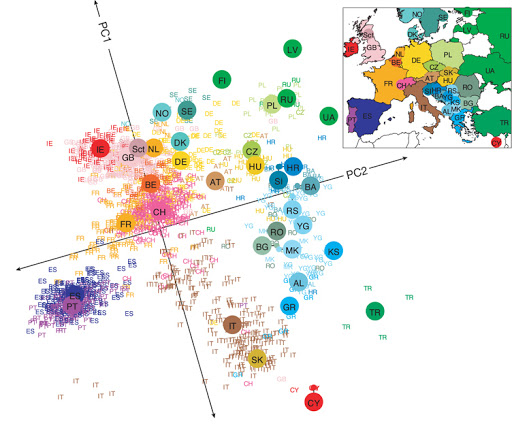# Lecture 19: Dimensionality Reduction¶

### Applied Machine Learning¶

Volodymyr Kuleshov
Cornell Tech

# Part 1: What is Dimensionality Reduction?¶

Dimensionality reduction is another important unsupervised learning problem with many applications.

We will start by defining the problem and providing some examples.

# Review: Unsupervised Learning¶

We have a dataset without labels. Our goal is to learn something interesting about the structure of the data:

• Clusters hidden in the dataset.
• Outliers: particularly unusual and/or interesting datapoints.
• Useful signal hidden in noise, e.g. human speech over a noisy phone.

# Dimensionality Reduction: Examples¶

Consider a dataset $\mathcal{D} = \{x^{(i)} \mid i = 1,2,...,n\}$ of motorcylces, characterized by a set of attributes.

• Attributes include size, color, maximum speed, etc.
• Suppose that two attributes are closely correlated: e.g., $x^{(i)}_j$ is the speed in mph and $x^{(i)}_k$ is the speed in km/h.
• The real dimensionality of the data is $d-1$!

We would like to automatically identify the right data dimensionality.

Another example can be obtained on the Iris flower dataset.

Consider the petal length and the petal width of the flowers: they are closely correlated.

This suggests that we may reduce the dimensionality of the problem to one dimension: petal size.

# Dimensionality Reduction¶

More generally, a dimensionality reduction algorithm learns from data an unsupervised model $$f_\theta : \mathcal{X} \to \mathcal{Z},$$ where $\mathcal{Z}$ is a low-dimensional representation of the data.

For each input $x^{(i)}$, $f_\theta$ computes a low-dimensional representation $z^{(i)}$.

# Linear Dimensionality Reduction¶

Suppose $\mathcal{X} = \mathbb{R}^d$ and $\mathcal{Z} = \mathbb{R}^p$ for some $p < d$. The transformation $$f_\theta : \mathcal{X} \to \mathcal{Z}$$ is a linear function with parameters $\theta = W \in \mathbb{R}^{d \times p}$ that is defined by $$z = f_\theta(x) = W^\top \cdot x.$$ The latent dimension $z$ is obtained from $x$ via a matrix $W$.

# Example: Discovering Structure in Digits¶

Dimensionality reduction can reveal interesting structure in digits without using labels.# Example: DNA Analysis¶

Even linear dimensionality reduction is powerful. Here, in uncovers the geography of European countries from only DNA data# Other Kinds of Dimensionality Reduction¶

We will focus on linear dimensionality reduction this lecture, but there exist many other methods:

• Non-linear methods based on kernels (e.g., Kernel PCA)
• Non-linear methods based on deep learning (e.g., variational autoencoders)
• Non-linear methods based on maximizing signal independence (independent component analysis)
• Probabilistic versions of the above

See the scikit-learn guide for more!# Part 2: Principal Component Analysis¶

We will now describe principal component analysis (PCA), one of the most widely used algorithms for dimensionality reduction.

# Components of an Unsupervised Learning Problem¶

At a high level, an unsupervised machine learning problem has the following structure:

$$\underbrace{\text{Dataset}}_\text{Attributes} + \underbrace{\text{Learning Algorithm}}_\text{Model Class + Objective + Optimizer } \to \text{Unsupervised Model}$$

The dataset $\mathcal{D} = \{x^{(i)} \mid i = 1,2,...,n\}$ does not include any labels.

# Review: Linear Dimensionality Reduction¶

Suppose $\mathcal{X} = \mathbb{R}^d$ and $\mathcal{Z} = \mathbb{R}^p$ for some $p < d$. The transformation $$f_\theta : \mathcal{X} \to \mathcal{Z}$$ is a linear function with parameters $\theta = W \in \mathbb{R}^{d \times p}$ that is defined by $$z = f_\theta(x) = W^\top x.$$ The latent dimension $z$ is obtained from $x$ via a matrix $W$.

# Principal Components Model¶

Principal component analysis (PCA) assumes that

• Datapoints $x \in \mathbb{R}^{d}$ live close to a low-dimensional subspace $\mathcal{Z} = \mathbb{R}^p$ of dimension $p<d$
• The subspace $\mathcal{Z} = \mathbb{R}^p$ is spanned by a set of orthonormal vectors $w^{(1)}, w^{(2)}, \ldots, w^{(p)}$
• The data $x$ are approximated by a linear combination $\tilde x$ of the $w^{(k)}$ $$x \approx \tilde x = \sum_{k=1}^p w^{(k)} z_k = W z$$ for some $z \in \mathcal{X}$ that are the coordinates of $\tilde x$ in the basis $W$.

In this example, the data lives in a lower-dimensional 2D plane within a 3D space (image credit).